1. /
2. CBSE
3. /
4. Class 08
5. /
6. Mathematics
7. /
8. NCERT Solutions for Class...

# NCERT Solutions for Class 8 Maths Exercise 5.2

NCERT solutions for Class 8 Maths Data Handling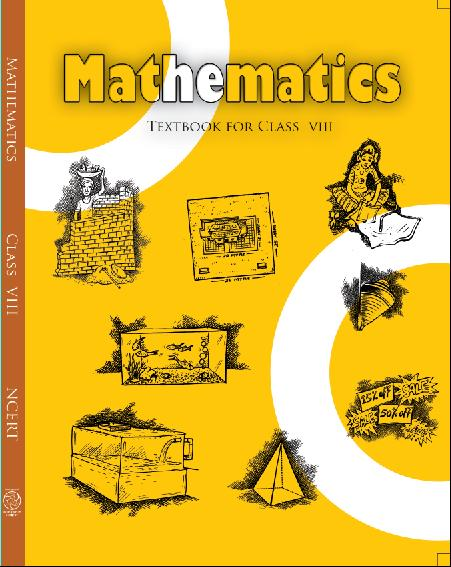## NCERT Solutions for Class 8 Maths Data Handling

###### 1. A survey was made to find the type of music that a certain group of young people liked in a city.

Adjoining pie chart shows the findings of this survey.

From this pie chart, answer the following:

(i) If 20 people liked classical music, how many young people were surveyed?

(ii) Which type of music is liked by the maximum number of people?

(iii) If a cassette company were to make 1000 CD’s, how many of each type would they make?

Ans. (i) 10% represents 100 people.

Therefore 20% represents =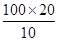= 200 people

Hence, 200 people were surveyed.

(ii) Light music is liked by the maximum number of people.

(iii) CD’s of classical music =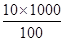= 100

CD’s of semi-classical music =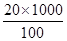= 200

CD’s of light music =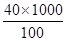= 400

CD’s of folk music =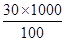= 300

NCERT Solutions for Class 8 Maths Exercise 5.2

###### 2. A group of 360 people were asked to vote for their favourite season from the three seasons rainy, winter and summer.

(i) Which season got the most votes?

(ii) Find the central angle of each sector.

(iii) Draw a pie chart to show this information.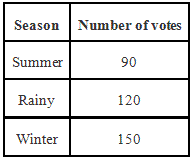Ans. (i) Winter season got the most votes.

(ii) Central angle of summer season =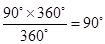Central angle of rainy season

=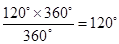Central angle of winter season

=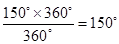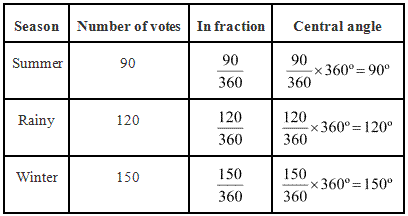(iii)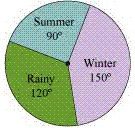NCERT Solutions for Class 8 Maths Exercise 5.2

###### 3. Draw a pie chart showing the following information. The table shows the colours preferred by a group of people.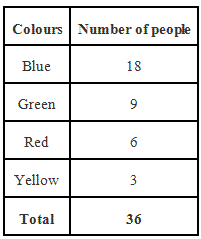Ans. Here, central angle =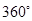and total number of people = 36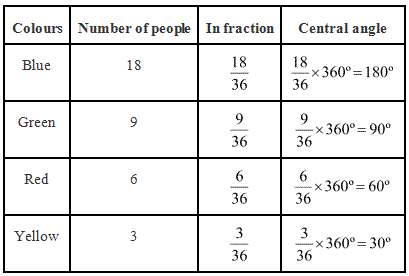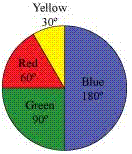NCERT Solutions for Class 8 Maths Exercise 5.2

###### 4. The adjoining pie chart gives the marks scored in an examination by a student in Hindi, English, Mathematics, Social Science and Science. If the total marks obtained by the students were 540, answer the following questions: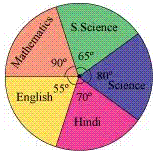(i) In which subject did the student score 105 marks?

(Hint: for 540 marks, the central angle =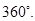So, for 105 marks, what is the central angle?)

(ii) How many more marks were obtained by the student in Mathematics than in Hindi?

(iii) Examine whether the sum of the marks obtained in Social Science and Mathematics is more than that in Science and Hindi.

(Hint: Just study the central angles)

Ans.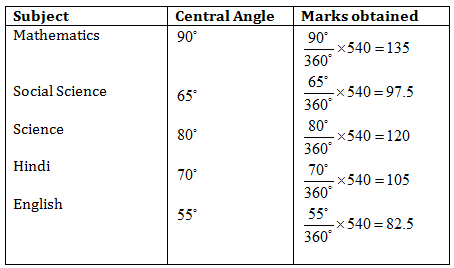(i) The student scored 105 marks in Hindi.

(ii) Marks obtained in Mathematics = 135

Marks obtained in Hindi = 105

Difference = 135 – 105 = 30

Thus, 30 more marks were obtained by the student in Mathematics than in Hindi.

(iii) The sum of marks in Social Science and Mathematics = 97.5 + 135 = 232.5

The sum of marks in Science and Hindi = 120 + 105 = 225

Yes, the sum of the marks in Social Science and Mathematics is more than that in Science and Hindi.

NCERT Solutions for Class 8 Maths Exercise 5.2

###### 5. The number of students in a hostel, speaking different languages is given below.

Display the data in a pie chart.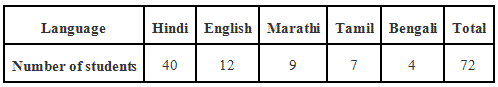Ans.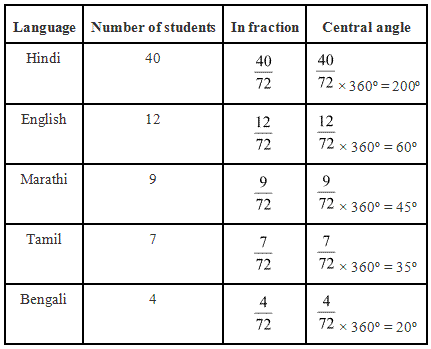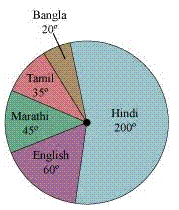Pie chart at above given data is as follows.

## NCERT Solutions for Class 8 Maths Exercise 5.2

NCERT Solutions Class 8 Mathematics PDF (Download) Free from myCBSEguide app and myCBSEguide website. Ncert solution class 8 Mathematics includes text book solutions from Class 8 Maths Book . NCERT Solutions for CBSE Class 8 Maths have total 16 chapters. 8 Maths NCERT Solutions in PDF for free Download on our website. Ncert class 8 solutions PDF and Maths ncert class 8 PDF solutions with latest modifications and as per the latest CBSE syllabus are only available in myCBSEguide.

## CBSE app for Class 8

To download NCERT Solutions for class 8 Social Science, Computer Science, Home Science,Hindi ,English, Maths Science do check myCBSEguide app or website. myCBSEguide provides sample papers with solution, test papers for chapter-wise practice, NCERT solutions, NCERT Exemplar solutions, quick revision notes for ready reference, CBSE guess papers and CBSE important question papers. Sample Paper all are made available through the best app for CBSE students and myCBSEguide website.Test Generator

Create question paper PDF and online tests with your own name & logo in minutes.myCBSEguide

Question Bank, Mock Tests, Exam Papers, NCERT Solutions, Sample Papers, Notes

### 3 thoughts on “NCERT Solutions for Class 8 Maths Exercise 5.2”

1. This is women full for five man

2. i like this i daily study with cbse guide com thank you so much

3. its very good.my choice is that you can also show how did that particular answer got?